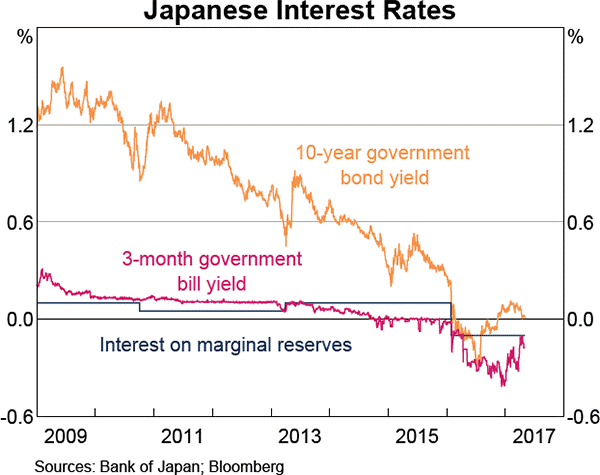# Fight Finance

#### CoursesTagsRandomAllRecentScores

In the below term structure of interest rates equation, all rates are effective annual yields and the numbers in subscript represent the years that the yields are measured over:

$$(1+r_{0-3})^3 = (1+r_{0-1})(1+r_{1-2})(1+r_{2-3})$$

Which of the following statements is NOT correct?

In the below term structure of interest rates equation, all rates are effective annual yields and the numbers in subscript represent the years that the yields are measured over:

$$(1+r_{0-3})^3 = (1+r_{0-1})(1+r_{1-2})(1+r_{2-3})$$

Which of the following statements is NOT correct?

Which of the following statements about yield curves is NOT correct?

Refer to the below graph when answering the questions.Which of the following statements is NOT correct?

Which of the following statements is NOT correct? An inverted US government bond yield curve indicates that: